Download PresentationChapter 5

# Chapter 5 - PowerPoint PPT Presentation

Chapter 5. INCOME AND SUBSTITUTION EFFECTS. Objectives. How will changes in prices and income influence influence consumer’s optimal choices? We will look at partial derivatives. Demand Functions (review). We have already seen how to obtain consumer’s optimal choiceI am the owner, or an agent authorized to act on behalf of the owner, of the copyrighted work described.
Download Presentation## Chapter 5

An Image/Link below is provided (as is) to download presentation

Download Policy: Content on the Website is provided to you AS IS for your information and personal use and may not be sold / licensed / shared on other websites without getting consent from its author.While downloading, if for some reason you are not able to download a presentation, the publisher may have deleted the file from their server.

- - - - - - - - - - - - - - - - - - - - - - - - - - E N D - - - - - - - - - - - - - - - - - - - - - - - - - -
Presentation Transcript### Chapter 5

INCOME AND SUBSTITUTION EFFECTSObjectives
• How will changes in prices and income influence influence consumer’s optimal choices?
• We will look at partial derivatives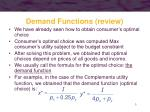Demand Functions (review)
• We have already seen how to obtain consumer’s optimal choice
• Consumer’s optimal choice was computed Max consumer’s utility subject to the budget constraint
• After solving this problem, we obtained that optimal choices depend on prices of all goods and income.
• We usually call the formula for the optimal choice: the demand function
• For example, in the case of the Complements utility function, we obtained that the demand function (optimal choice) is:Demand Functions
• If we work with a generic utility function (we do not know its mathematical formula), then we express the demand function as:

x* = x(px,py,I)

y* = y(px,py,I)

• We will keep assuming that prices and income is exogenous, that is:
• the individual has no control over these parameters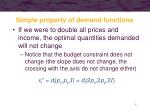Simple property of demand functions
• If we were to double all prices and income, the optimal quantities demanded will not change
• Notice that the budget constraint does not change (the slope does not change, the crossing with the axis do not change either)

xi* = di(px,py,I) = di(2px,2py,2I)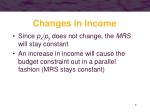Changes in Income
• Since px/py does not change, the MRS will stay constant
• An increase in income will cause the budget constraint out in a parallel fashion (MRS stays constant)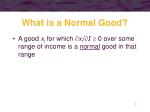What is a Normal Good?
• A good xi for which xi/I 0 over some range of income is a normal good in that range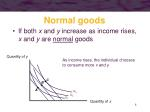C

B

A

U3

U2

U1

Normal goods
• If both x and y increase as income rises, x and y are normal goods

Quantity of y

As income rises, the individual chooses

to consume more x and y

Quantity of xWhat is an inferior Good?
• A good xi for which xi/I< 0 over some range of income is an inferior good in that range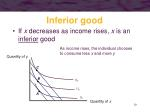C

B

U3

U2

A

U1

Inferior good
• If x decreases as income rises, x is an inferior good

As income rises, the individual chooses

to consume less x and more y

Quantity of y

Quantity of xChanges in a Good’s Price
• A change in the price of a good alters the slope of the budget constraint (px/py)
• Consequently, it changes the MRS at the consumer’s utility-maximizing choices
• When a price changes, we can decompose consumer’s reaction in two effects:
• substitution effect
• income effectSubstitution and Income effects
• Even if the individual remained on the same indifference curve when the price changes, his optimal choice will change because the MRS must equal the new price ratio
• the substitution effect
• The price change alters the individual’s real income and therefore he must move to a new indifference curve
• the income effectSign of substitution effect (SE)

SE is always negative, that is, if price increases, the substitution effect makes quantity to decrease and conversely. See why:

1) Assume px decreases, so: px1< px0

2) MRS(x0,y0)= px0/ py0 & MRS(x1,y1)= px1/ py0

1 and 2 implies that:

MRS(x1,y1)<MRS(x0,y0)

As the MRS is decreasing in x, this means that x has increased, that is: x1>x0Suppose the consumer is maximizing

utility at point A.

If the price of good x falls, the consumer

will maximize utility at point B.

B

A

U2

U1

Total increase in x

Changes in the optimal choice when a price decreases

Quantity of y

Quantity of xTo isolate the substitution effect, we hold

utility constant but allow the

relative price of good x to change.

Purple is parallel to the new one

The substitution effect is the movement

from point A to point C

C

Substitution effect

Substitution effect when a price decreases

Quantity of y

The individual substitutes

good x for good y

because it is now

relatively cheaper

A

U1

Quantity of xThe income effect is the movement

from point C to point B

B

Income effect

Income effect when the price decreases

The income effect occurs because the

individual’s “real” income changes

(hence utility changes) when

the price of good x changes

Quantity of y

If x is a normal good,

the individual will buy

more because “real”

income increased

A

C

U2

U1

Quantity of x

How would the graph change if the good was inferior?The substitution effect is the

movement from point A to point C

C

The income effect is the

movement from point C

to point B

Substitution effect

Income effect

Subs and income effects when a price increases

Quantity of y

An increase in the price of good x means that

the budget constraint gets steeper

A

B

U1

U2

Quantity of x

How would the graph change if the good was inferior?Price Changes forNormal Goods
• If a good is normal, substitution and income effects reinforce one another
• when price falls, both effects lead to a rise in quantity demanded
• when price rises, both effects lead to a drop in quantity demandedPrice Changes forInferior Goods
• If a good is inferior, substitution and income effects move in opposite directions
• The combined effect is indeterminate
• when price rises, the substitution effect leads to a drop in quantity demanded, but the income effect is opposite
• when price falls, the substitution effect leads to a rise in quantity demanded, but the income effect is opposite• If the income effect of a price change is strong enough, there could be a positive relationship between price and quantity demanded
• an increase in price leads to a drop in real income
• since the good is inferior, a drop in income causes quantity demanded to riseA Summary
• Utility maximization implies that (for normal goods) a fall in price leads to an increase in quantity demanded
• the substitution effect causes more to be purchased as the individual moves along an indifference curve
• the income effect causes more to be purchased because the resulting rise in purchasing power allows the individual to move to a higher indifference curve
• Obvious relation hold for a rise in price…A Summary
• Utility maximization implies that (for inferior goods) no definite prediction can be made for changes in price
• the substitution effect and income effect move in opposite directions
• if the income effect outweighs the substitution effect, we have a case of Giffen’s paradoxCompensated Demand Functions
• This is a new concept
• It is the solution to the following problem:
• MIN PXX+ PYY
• SUBJECT TO U(X,Y)=U0
• Basically, the compensated demand functions are the solution to the Expenditure Minimization problem that we saw in the previous chapter
• After solving this problem, we obtained that optimal choices depend on prices of all goods and utility. We usually call the formula: the compensated demand function
• x* = xc(px,py,U),
• y* = yc(px,py,U)Compensated Demand Functions
• xc(px,py,U0), and yc(px,py,U0) tell us what quantities of x and y minimize the expenditure required to achieve utility level U0 at current prices px,py
• Notice that the following relation must hold:
• pxxc(px,py,U0)+ pyyc(px,py,U0)=E(px,py,U0)
• So this is another way of computing the expenditure function !!!!Compensated Demand Functions
• There are two mathematical tricks to obtain the compensated demand function without the need to solve the problem:
• MIN PXX+ PYY
• SUBJECT TO U(X,Y)=U0
• One trick(A) (called Shephard’s Lemma) is using the derivative of the expenditure function
• Another trick(B) is to use the marshallian demand and the expenditure functionCompensated Demand Functions
• Sheppard’s Lema to obtain the compensated demand function

Intuition: a £1 increase in px raises necessary expenditures by x pounds, because £1 must be paid for each unit of x purchased.

Proof: footnote 5 in page 137Trick (B) to obtain compensated demand functions
• Suppose that utility is given by

utility = U(x,y) = x0.5y0.5

• The Marshallian demand functions are

x = I/2pxy = I/2py

• The expenditure function isAnother trick to obtain compensated demand functions

• Substitute the expenditure function into the Marshallian demand functions, and find the compensated ones:Compensated Demand Functions
• Demand now depends on utility (V) rather than income
• Increases in px changes the amount of x demanded, keeping utility V constant. Hence the compensated demand function only includes the substitution effect but not the income effectRoy’s identity
• It is the relation between marshallian demand function and indirect utility function

Proof of the Roy’s identity…Demand curves…
• We will start to talk about demand curves. Notice that they are not the same that demand functions !!!!The Marshallian Demand Curve
• An individual’s demand for x depends on preferences, all prices, and income:

x* = x(px,py,I)

• It may be convenient to graph the individual’s demand for x assuming that income and the price of y (py) are held constant…quantity of x

demanded rises.

px’

px’’

px’’’

U3

x

U2

U1

x1

x2

x3

x’

x’’

x’’’

I = px’ + py

I = px’’ + py

I = px’’’ + py

The Marshallian Demand Curve

Quantity of y

As the price

of x falls...

px

Quantity of x

Quantity of xThe Marshallian Demand Curve
• The Marshallian demand curve shows the relationship between the price of a good and the quantity of that good purchased by an individual assuming that all other determinants of demand are held constant
• Notice that demand curve and demand function is not the same thing!!!Shifts in the Demand Curve
• Three factors are held constant when a demand curve is derived
• income
• prices of other goods (py)
• the individual’s preferences
• If any of these factors change, the demand curve will shift to a new positionShifts in the Demand Curve
• A movement along a given demand curve is caused by a change in the price of the good
• a change in quantity demanded
• A shift in the demand curve is caused by changes in income, prices of other goods, or preferences
• a change in demandCompensated Demand Curves
• An alternative approach holds utility constant while examining reactions to changes in px
• the effects of the price change are “compensated” with income so as to constrain the individual to remain on the same indifference curve
• reactions to price changes include only substitution effects (utility is kept constant)Marshallian Demand Curves
• The actual level of utility varies along the demand curve
• As the price of x falls, the individual moves to higher indifference curves
• it is assumed that nominal income is held constant as the demand curve is derived
• this means that “real” income rises as the price of x fallsCompensated Demand Curves
• A compensated (Hicksian) demand curve shows the relationship between the price of a good and the quantity purchased assuming that other prices and utility are held constant
• The compensated demand curve is a two-dimensional representation of the compensated demand function

x* = xc(px,py,U)…quantity demanded

rises.

px’

px’’

px’’’

xc

U2

x’

x’’

x’’’

x’

x’’

x’’’

Compensated Demand Curves

Holding utility constant, as price falls...

Quantity of y

px

Quantity of x

Quantity of xAt px’’, the curves intersect because

the individual’s income is just sufficient to attain utility level U2

px’’

x’’

Compensated & Uncompensated Demand for normal goods

px

x

xc

Quantity of xAt prices above p’’x, income compensation is positive because the individual needs some help to remain on U2

px’

x’

x*

Compensated & Uncompensated Demand for normal goods

px

px’’

x

xc

Quantity of x

As we are looking at normal goods, income and substitution effects go in the same direction, so they are reinforced. X includes both while Xc only the substitution effect. That is what drives the relative position of both curvesAt prices below px2, income compensation is negative to prevent an increase in utility from a lower price

px’’’

x***

x’’’

Compensated & Uncompensated Demand for normal goods

px

px’’

x

xc

As we are looking at normal goods, income and substitution effects go in the same direction, so they are reinforced. X includes both while Xc only the substitution effect. That is what drives the relative position of both curves

Quantity of xCompensated & Uncompensated Demand
• For a normal good, the compensated demand curve is less responsive to price changes than is the uncompensated demand curve
• the uncompensated demand curve reflects both income and substitution effects
• the compensated demand curve reflects only substitution effectsRelations to keep in mind
• Sheppard’s Lema & Roy’s identity
• V(px,py,E(px,py,Uo)) = U0
• E(px,py,V(px,py,I0)) = I0
• xc(px,py,U0)=x(px,py,I0)A Mathematical Examination of a Change in Price
• Our goal is to examine how purchases of good x change when px changes

x/px

• Differentiation of the first-order conditions from utility maximization can be performed to solve for this derivativeA Mathematical Examination of a Change in Price
• However, for our purpose, we will use an indirect approach
• Remember the expenditure function

minimum expenditure = E(px,py,U)

• Then, by definition

xc(px,py,U) = x[px,py,E(px,py,U)]

• quantity demanded is equal for both demand functions when income is exactly what is needed to attain the required utility levelA Mathematical Examination of a Change in Price
• We can differentiate the compensated demand function and get

xc(px,py,U) = x[px,py,E(px,py,U)]A Mathematical Examination of a Change in Price
• The first term is the slope of the compensated demand curve
• the mathematical representation of the substitution effectA Mathematical Examination of a Change in Price
• The second term measures the way in which changes in px affect the demand for x through changes in purchasing power
• the mathematical representation of the income effectThe Slutsky Equation
• The substitution effect can be written as
• The income effect can be written asThe Slutsky Equation
• A price change can be represented byThe Slutsky Equation
• The first term is the substitution effect
• always negative as long as MRS is diminishing
• the slope of the compensated demand curve must be negativeThe Slutsky Equation
• The second term is the income effect
• if x is a normal good, then x/I > 0
• the entire income effect is negative
• if x is an inferior good, then x/I < 0
• the entire income effect is positiveA Slutsky Decomposition
• We can demonstrate the decomposition of a price effect using the Cobb-Douglas example studied earlier
• The Marshallian demand function for good x wasA Slutsky Decomposition
• The Hicksian (compensated) demand function for good x was
• The overall effect of a price change on the demand for x isA Slutsky Decomposition
• This total effect is the sum of the two effects that Slutsky identified
• The substitution effect is found by differentiating the compensated demand functionA Slutsky Decomposition
• We can substitute in for the indirect utility function (V)A Slutsky Decomposition
• Calculation of the income effect is easier
• By adding up substitution and income effect, we will obtain the overall effect